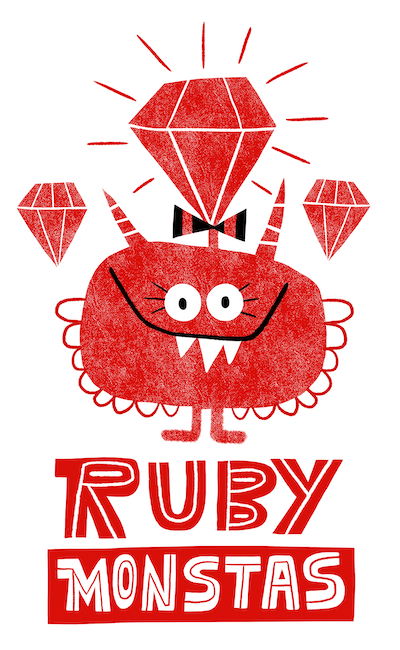# Ruby Monstas

Numbers## Numbers

Programming languages often have more than one number type

Ruby distinguishes:

• Integers (whole numbers)
• Floats (floating point numbers, decimal numbers)

## Integers

Example integers:

`-1353`
,
`-8`
,
`0`
,
`4`
,
`78`

Often used for counting things

## Floats

Example floats:

`-514.3556`
,
`-7.0`
,
`0.0`
,
`9.1567`
,
`16789.4212357`

Often used for calculations

## Integer calculations

Try these in IRB!

``7 + 5``
`=> 12`
``12 - 17``
`=> -5`
``5 * 9``
`=> 45`
``1 / 2``
`=> 0`

Wait a second, what?!

## Integer division

When dividing two integers, Ruby rounds the result down to the next integer!

The logic: If you put integers in, you want integers out, no matter what!

Try these in IRB!

``1.0 / 2``
`=> 0.5`
``1 / 2.0``
`=> 0.5`